# R语言线性回归和时间序列分析北京房价影响因素可视化案例

## 最近我们被客户要求撰写关于北京房价的研究报告。房价有关的数据可能反映了中国近年来的变化：

• 人们得到更多的资源（薪水），期望有更好的房子
• 人口众多
• 独生子女政策：如何影响房子的几何结构？更多的卧室，更多的空间

# 目的

• 数据准备：将数值特征转换为分类；缺失值
• EDA:对于数值特征和分类特征:平均价格与这些特征的表现
• 建模：
• 分割训练/测试给定年份的数据：例如，在2000年分割数据；根据这些数据训练回归模型
• 然后，在2016年之前的所有年里，预测每套房子的价值。
• 用于验证的度量将是房屋的平均价格（即每年从测试样本中获得平均价格和预测值）

# 数据准备

• `url：获取数据（字符）的url`
• `id:id（字符）`
• `Lng：和Lat坐标，使用BD09协议。（数字）`
• `Cid：社区id（数字）`
• `交易时间：交易时间（字符）`
• `DOM：市场活跃日。（数字）`
• `关注者：交易后的人数。（数字）`
• `总价：（数值）`
• `价格：按平方计算的平均价格（数值）`
• `面积：房屋的平方（数字）`
• 起居室`：客厅数（字符）`
• 客厅`：客厅数（字符）`
• `厨房：厨房数量（数字）`
• `浴室数量（字符）`
• `房子的高度`
• `建筑类型：包括塔楼（1）、平房（2）、板塔组合（3）、板（4）（数值）`
• `施工时间`
• `装修：包括其他（1）、粗（2）、简单（3）、精装（4）（数值）`
• `建筑结构：包括未清（1）、混合（2）、砖和木（3）、砖混凝土（4）、钢（5）和钢-混凝土复合材料（6）。（数值）`
• `梯梯比：同层居民数与电梯数量的比例。`
• `电梯有（1）或没有电梯（0）（数值）`
• `五年期：业主拥有不到5年的财产（数字）`

## 数据清理、特征创建

• 从网址上，我发现它有位置信息，如chengjiao/101084782030。同样，一个简单的regexp进行省特征提取。
• 另一个大的数据准备工作是转换一些数字特征，比如地铁，地铁站附近的房子编码为1，相反的情况编码为0。
• 还有很大一部分DOM缺失。我既不能在建模中使用这个特性，也不能删除NA，但它也会减小数据帧的大小。
`````` #从网址中提取省份
sapply(df\$url, function(x) strsplit(x,'/')[]) ``````

### 检查缺失

``````#缺失数据图

ggplot(data = .,aes(x = V2, y = V1)) + geom_tile(aes(fill = value )) + ``````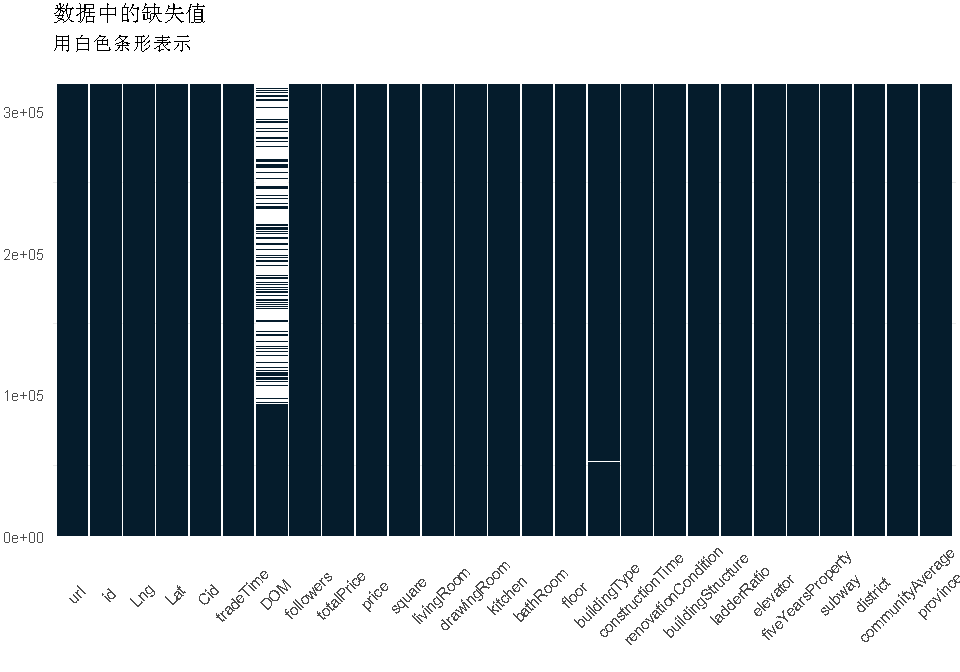• 如上所述，DOM的很大一部分丢失了。我决定先保留这个特性，然后用中间值来填充缺失的值（分布是非常倾斜的）
• 否则，buildingType和communityAverage（pop.）中只有几个缺少的值，我决定简单地删除这些值。事实上，它们只占了约30行，而整个数据集的数据量为300k+，因此损失不会太大。
• 下面我简单地删除了我以后不打算使用的特征。
`` ifelse(is.na(df\$DOM),median(df\$DOM,na.rm=T),df\$DOM) ``

## 用于将数字转换为类别的自定义函数

``df2\$livingRoom <- as.numeric(df2\$livingRoom) ``

`````` df2\$renovationCondition <- sapply(df2\$renovationCondition, ionCondition)

df2\$buildingStructure <- sapply(df2\$buildingStructure, makeStructure)
df2\$elevator <- ifelse(df2\$elevator==1,'has_elevator','no_elevator') ``````

### 缺失值检察

``````# 缺失数据图
df2 %>% is.na %>% melt %>%
ggplot(data = .,aes(x = Var2, y = Var1)) + geom_tile(aes(fill = value)) +
scale_fill_manual(values = c("grey20","white")) + theme_minimal(14) + ``````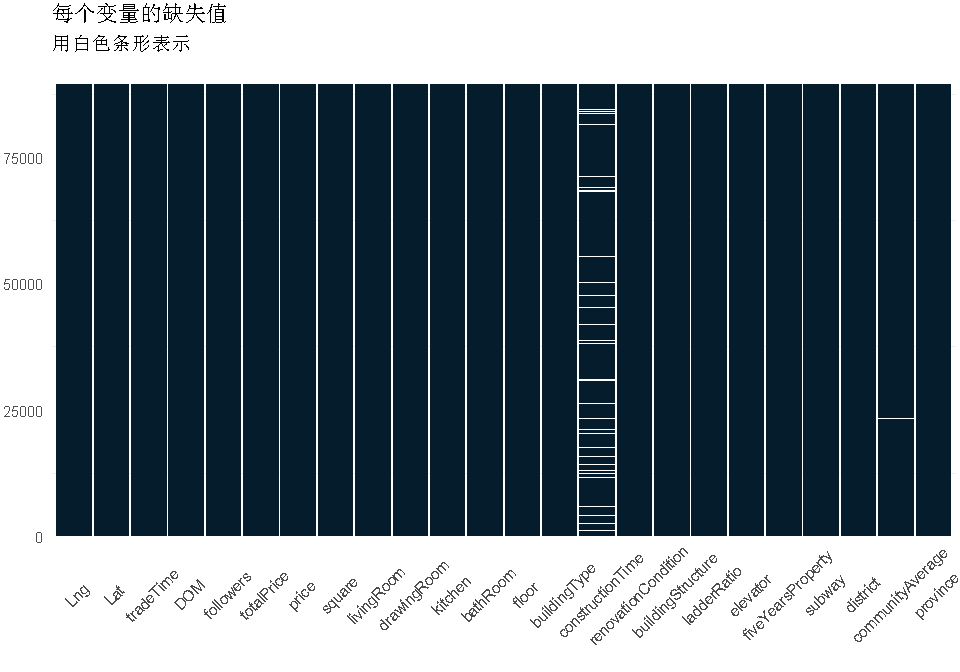``kable(df %>% group_by(constructionTime) %>% summarise(count=n()) %>% arrange(-count) %>% head(5))``

# R语言用线性回归模型预测空气质量臭氧数据

``df3 <- data.frame(df2 %>% na.omit())``

# 插补后的最终检查

``any(is.na(df3))``

### 随时关注您喜欢的主题

``##  FALSE``

# 探索性分析

• 数值：相关矩阵
• 分类：箱线图和地图

totalPrice将是回归模型的目标变量。

## 数值特征

``````corrplot(cor(
df3  ,
tl.col='black')``````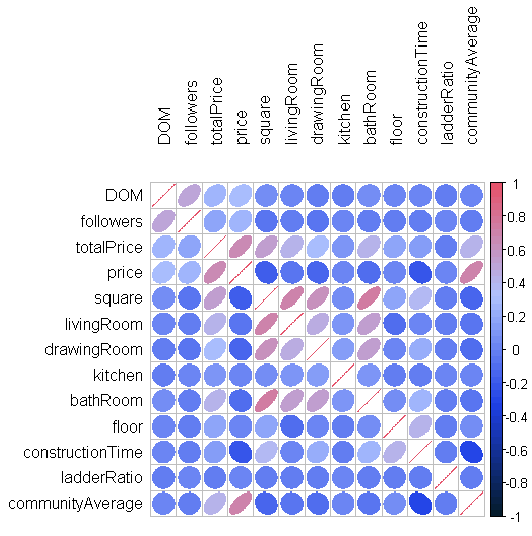• totalPrice与communityAverage有很强的正相关关系，即人口密集区的房价较高
• totalPrice与客厅、卫浴室数量有一定的正相关关系。
• 至于面积变量，我们看到它与上述变量也有很强的相关性：这是有道理的，因为如果房子的面积大，可以建造更多的房间（显而易见）。
• 其他一些有趣的相关性：communityAverage与建筑时间呈负相关，这意味着在人口密集区建房所需的时间更短

## 分类特征

### 地图

• 中国三级（省）地图
• 我看了看城郊，它位于北京附近，所以我过滤了那个特定省份的地图
``````ggplot() +
geom_polygon(data = shapefile_test,aes(x = long, y = lat, group = group),

BeijingLoc <- data.frame('Long'=116.4075,'Lat' = 39.904)``````

### 建筑结构

``makeEDA('buildingStructure' )``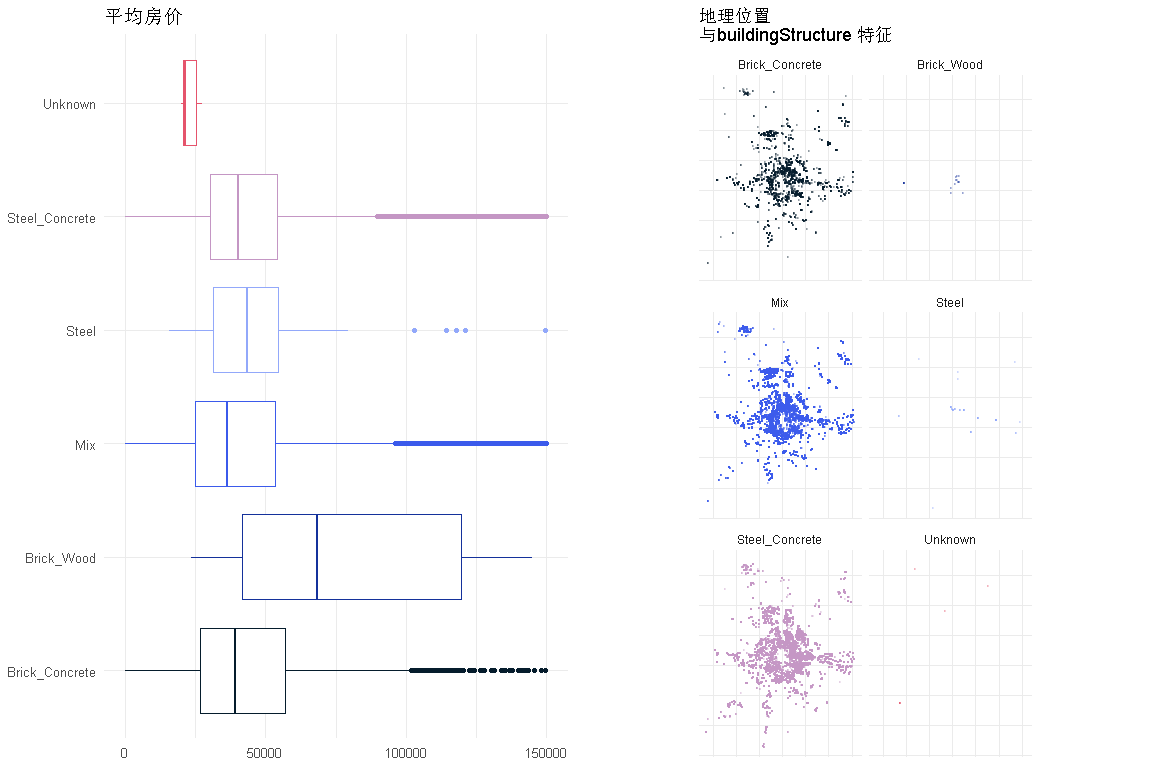# 砖木结构的房屋是最昂贵的，几乎是其他类型房屋的两倍

### 建筑类型

``makeEDA('buildingType' )``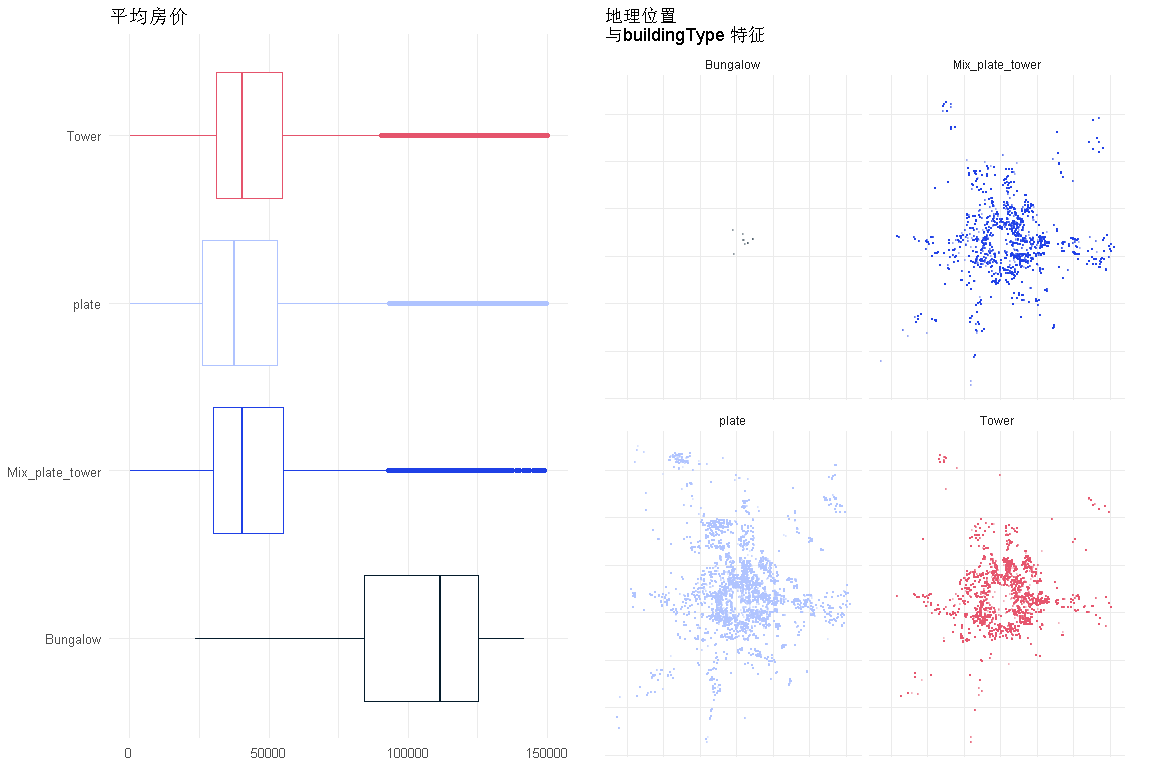• `平房是最昂贵的，也是最本地化的`

### `装修条件`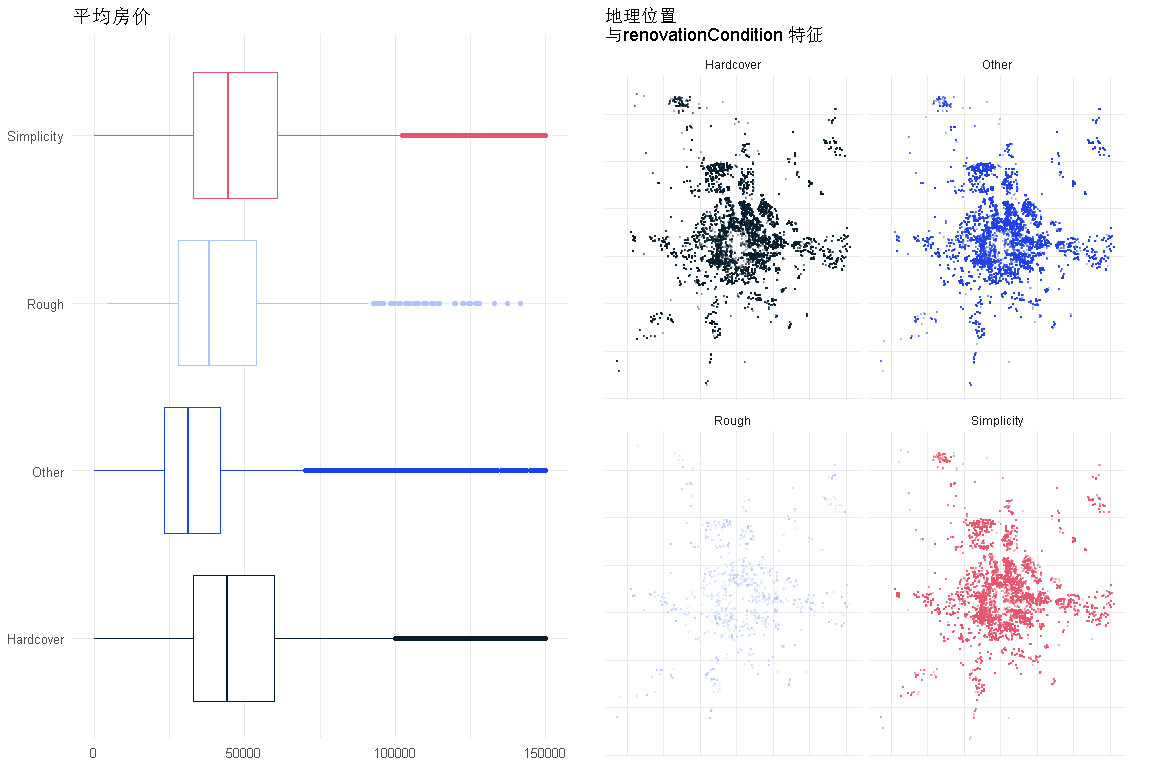### `电梯`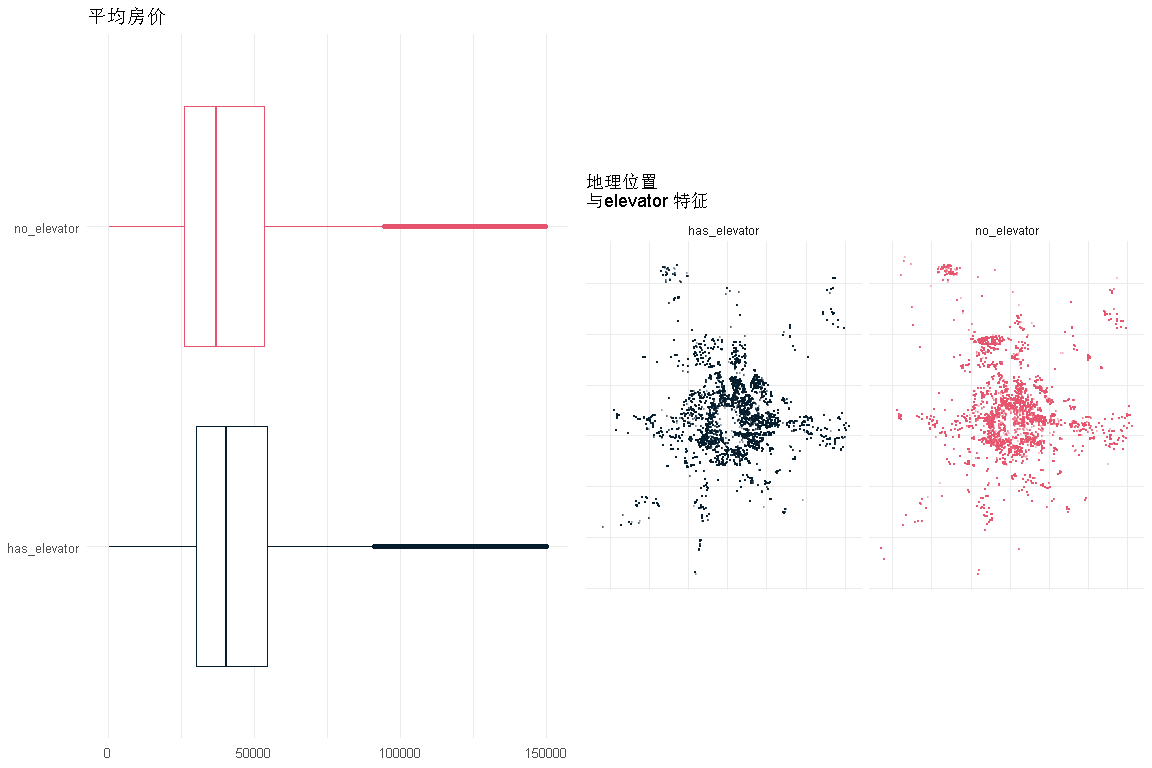• 价格对电梯的依赖性非常小
• 住宅的分布与这一特征是相对相等的。

### `地铁`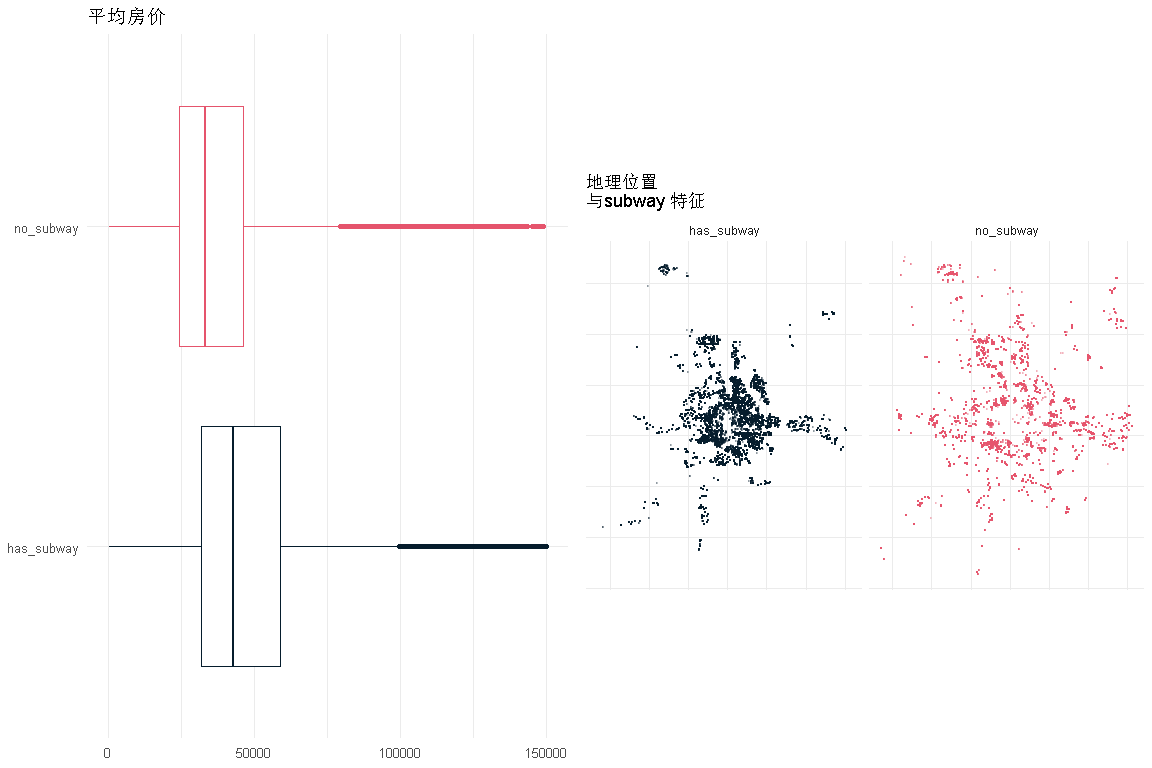• 价格对地铁站附近的依赖性非常小。
• 住宅的分布与这一特征是相对相等的。

### 是否满_五年_

``makeFeatureCatEDA('fiveYearsProperty', length(unique(df3\$fiveYearsProperty)))``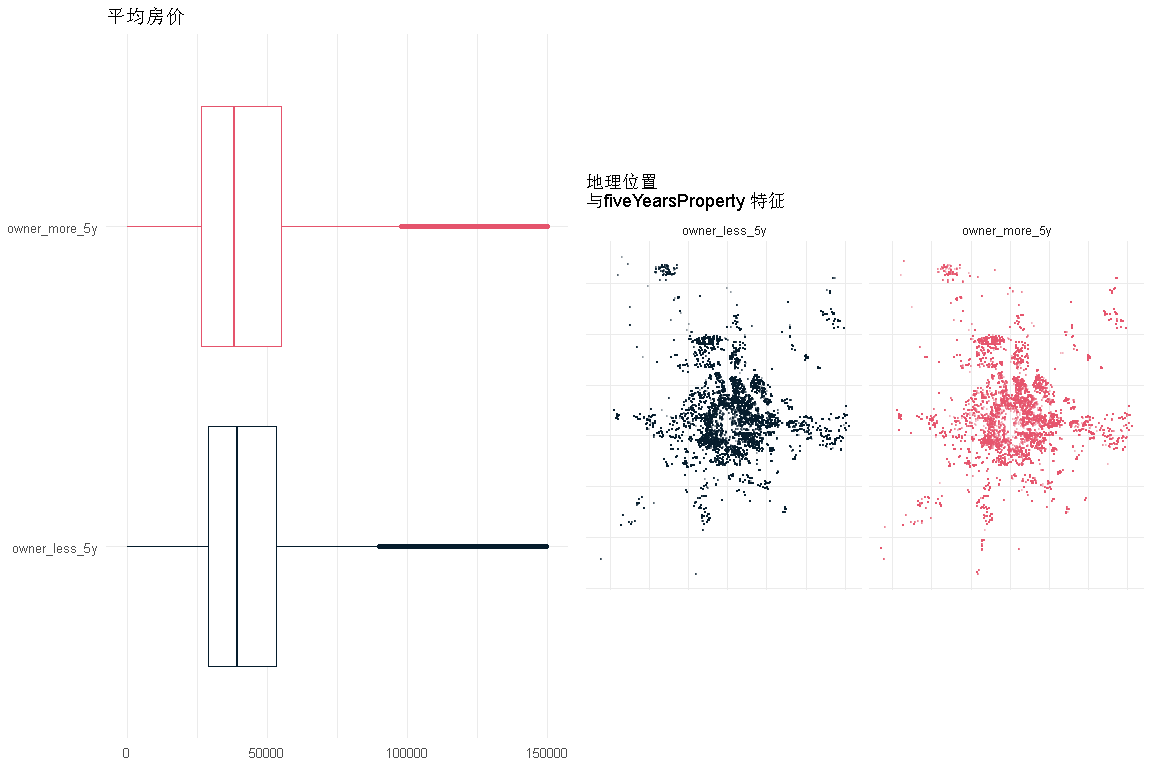• 对于是否拥有不到5年房产来说，价格的依赖性确实很小
• 就这一特征而言，房子的分布是相对平等的

### `区域`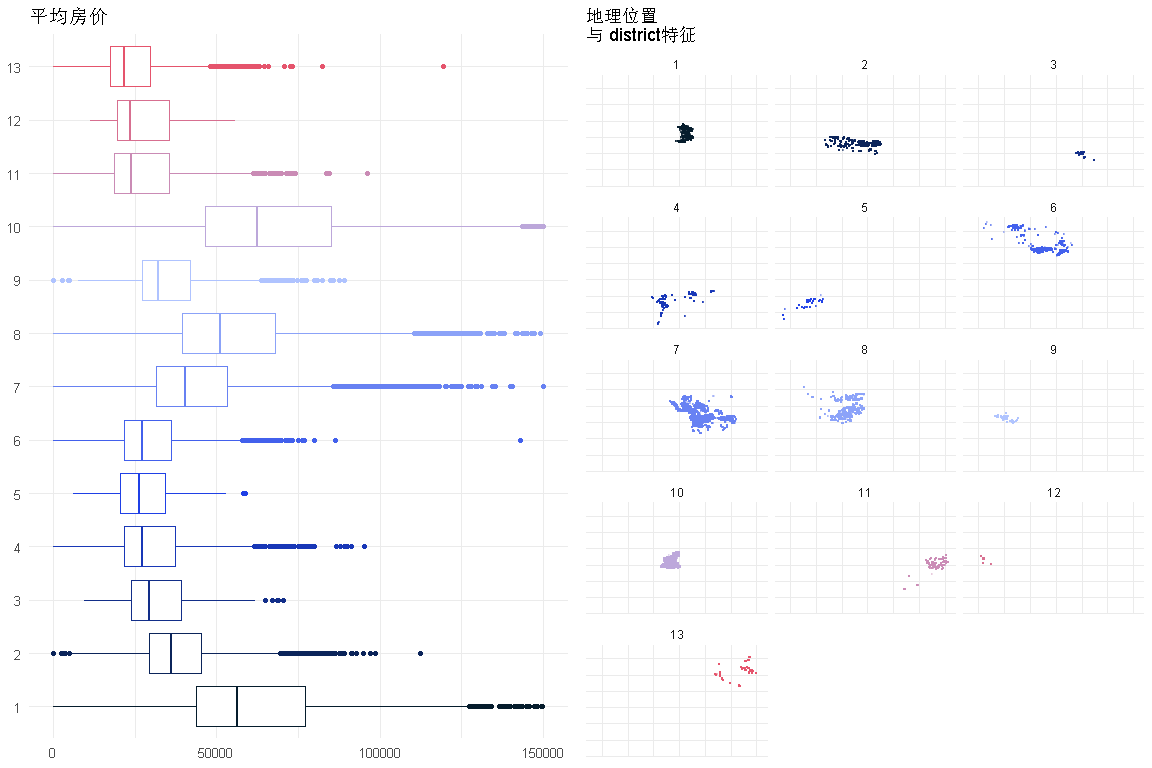# 回归模型

## 策略

• `按年度和月份分组，得到房屋的数量和均价`
• 拆分数据集：
• 对于年[2010-2017]=在这组年上训练并运行回归模型
• 对于>2017年：逐月对测试样本并预测平均价格

# 首先需要看看我们想要预测什么

``````df3\$year <- year(df3\$tradeTimeTs)
``````df3 %>% filter(year>2009) %>% group_by(monthlyTrad) %>%
summarise(count=n(), mean = mean(price)) %>%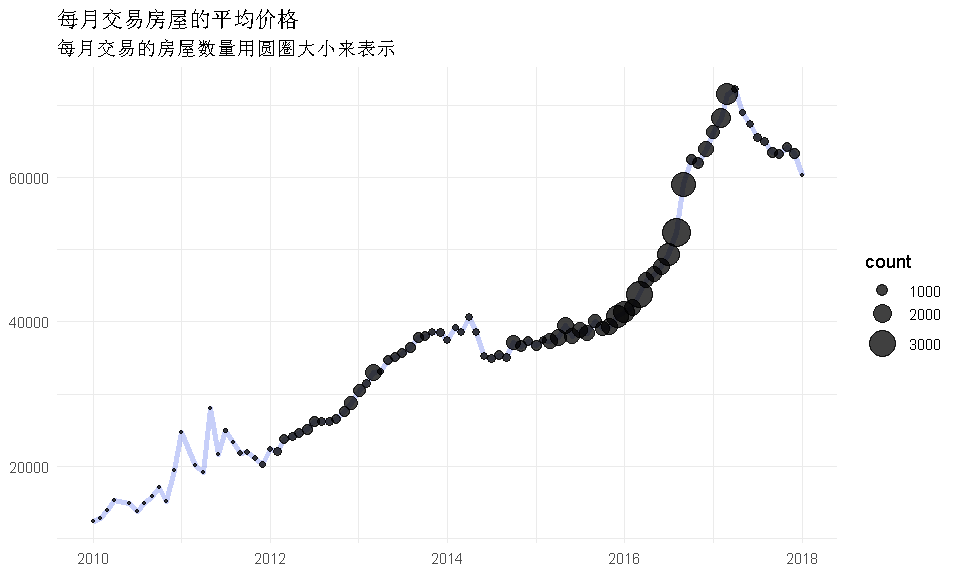• 平均价格上涨至2017年中期，然后迅速下降
• 同时，房屋数量随着价格的上涨而增加，而且现在房屋交易的数量也随着价格的上涨而减少。

## 准备训练/测试样本

``````df_train <- data.frame(df  %>% filter(year>2009 & year<2017))
df_test <- data.frame(df %>% filter(year>=2017))
as.data.frame(cbind(
df_train %>% select_if(is.numeric) %>% select(-Lng, -Lat, -year, -month),
'bldgType'= dummy.code(df_train\$buildingType),
'bldgStruc'= dummy.code(df_train\$buildingStructure),
'renovation'= dummy.code(df_train\$renovationCondition),
'hasElevator'= dummy.code(df_train\$elevator), ``````

``````regressors<-c('lm')

Control <- trainControl(method = "cv",number = 5, repeats=3)
for(r in regressors){
cnt<-cnt+1
res[[cnt]]<-train(totalPrice ~., data = train ,method=r,trControl =  Control) ``````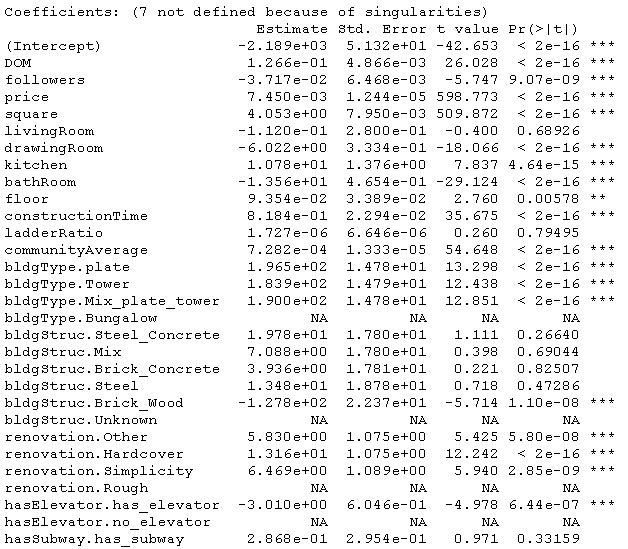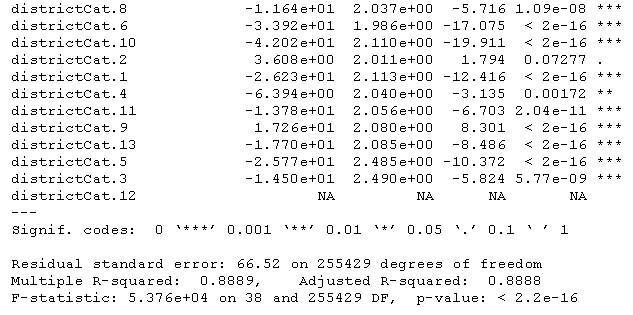r^2在0.88左右，不错。让我们看看细节。

### 训练精度

`````` g1<-ggplot(data=PRED,aes(x=Prediction,y=True)) + geom_jitter() + geom_smooth(method='lm',size=.5) +
#计算指标
mse <- mean((PRED\$True-PRED\$Prediction)^2)
rmse<-mse^0.5
SSE = sum((PRED\$Pred - PR ``````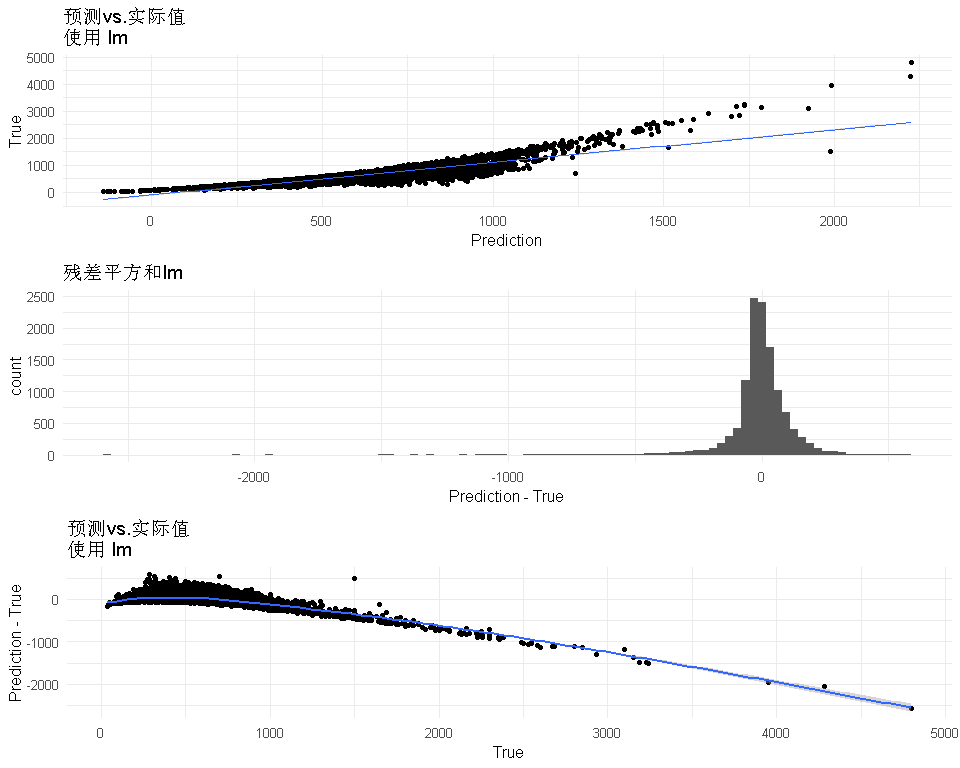``##  "MSE: 15952.845934 RMSE : 126.304576 R2 :0.795874"``
• 所以看起来残差还不错（分布是正态的，以0为中心），但对于低价格来说似乎失败了。训练和测试样本的预测与时间的关系

• 基本上与上述相同，但我将重复预测所有月份的训练数据
• 我的目标指标是平均房价。
• 训练是在10多年的训练样本中完成的，因此逐月查看预测将非常有趣。
`````` # 训练样本->训练精度

for (i in 1:length(dates_train)){
current_df <- prepareDF(current_df)
current_pred <- mean(predict(res[],current_df))

#运行测试样本-->测试精度

for (i in 1:length(dates_test)){
current_df <- prepareDF(current_df)
current_pred <- mean(predict(res[],current_df)) ``````
`````` RES %>% reshape2::melt(id=c('date','split')) %>%
ggplot(aes(x=date,y=value)) + geom_line(aes(color=variable, lty=split),size=1) + ``````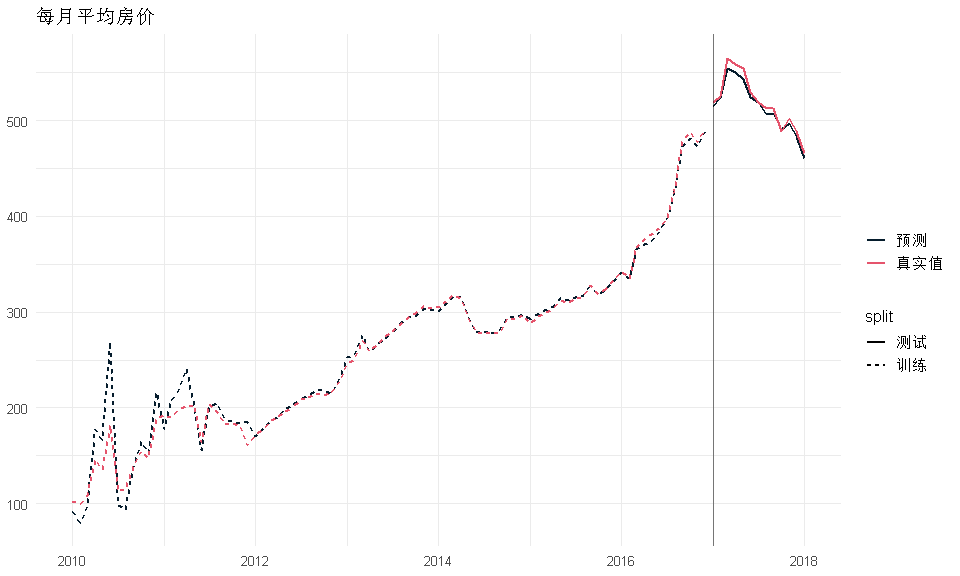• 预测对于2012年之后的数据确实非常好，这可能与有足够数据的月份相对应

# 改进

## 地理位置作为特征

• 下面是一个有趣的图；它显示了每个位置的总价格。 在二维分布的中心，价格更高。
• 这个想法是计算每个房子到中心的距离，并关联一个等级/分数
``````BeijingLoc <- data.frame('Long'=116.4075,'Lat' = 39.904)
df3 %>% ggplot(aes(x=Lng,y=Lat)) + geom_point(aes(color=price),size=.1,alpha=.5)  +
theme(legend.position = 'bottom') + ``````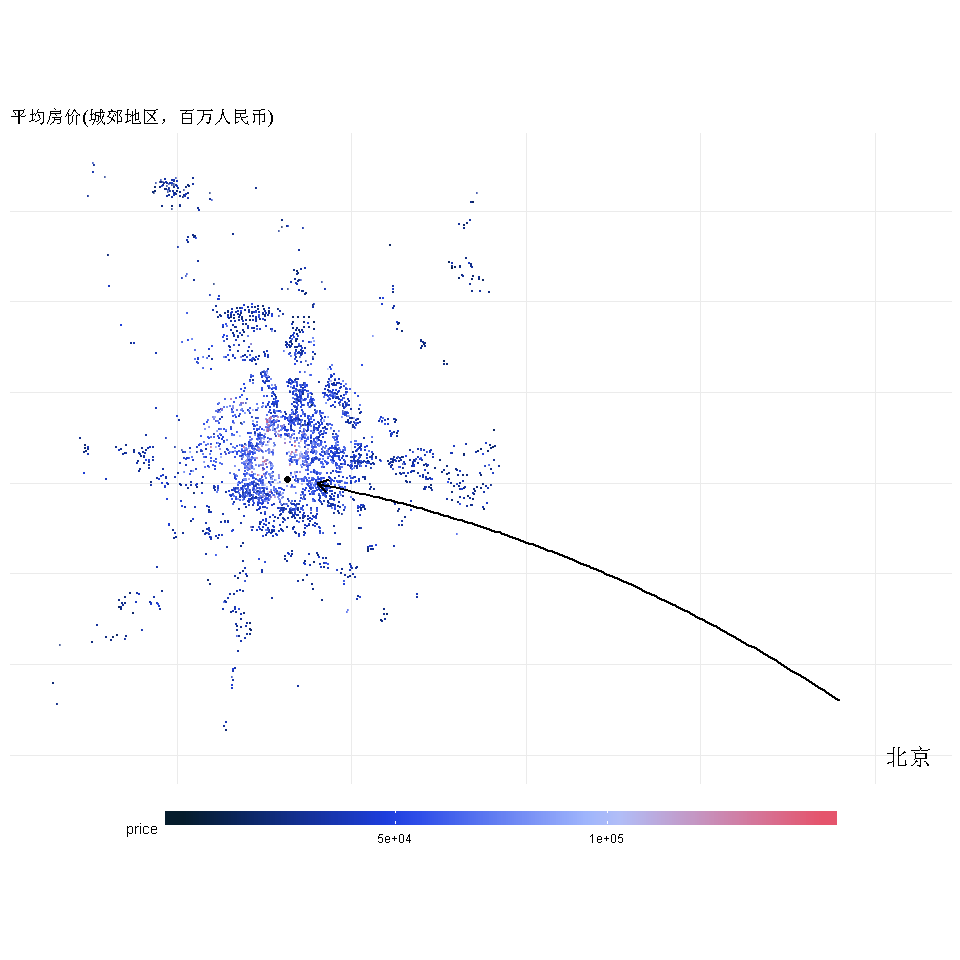Kaizong Ye拓端研究室（TRL）的研究员。

​非常感谢您阅读本文，如需帮助请联系我们！QQ在线咨询

15121130882

0571-63341498

## 关注有关新文章的微信公众号

This will close in 0 seconds